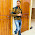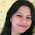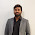C code to Encrypt Message using PlayFair (Monarchy) Cipher

C program to implement PlayFair Cipher to encrypt a given message.

#include<stdio.h>
#include<conio.h>
void main()
{
char v,w,ch,string,arr,key,a,b,enc;
int temp,i,j,k,l,r1,r2,c1,c2,t,var;
FILE * fp;
fp=fopen("sk.txt","r");   //keep message in sk.txt (e.g. jamia)
clrscr();
printf("Enter the key\n");
fflush(stdin);
scanf("%s",&key);
l=0;
while(1)
{
ch=fgetc(fp);
if(ch!=EOF)
{
string[l++]=ch;
}
if(ch==EOF)
break;
}
string[l]='\0';
puts(string);
for(i=0;key[i]!='\0';i++)
{
for(j=i+1;key[j]!='\0';j++)
{
if(key[i]==key[j])
{
temp=1;
break;
}
}
}
if(temp==1)
printf("invalid key");
else
{
k=0;
a='a';
//printf("%c",b);
for(i=0;i<5;i++)
{
for(j=0;j<5;j++)
{
if(k<strlen(key))
arr[i][j]=key[k];
else if(k==strlen(key))
{
b:
for(l=0;l<strlen(key);l++)
{
if(key[l]==a)
{
a++;
goto b;
}
}
arr[i][j]=a;
if(a=='i')
a=a+2;
else
a++;
}
if(k<strlen(key))
k++;
}
}
printf("\n");
printf("The matrix is\n");
for(i=0;i<5;i++)
{
for(j=0;j<5;j++)
{
printf("%c",arr[i][j]);
}
printf("\n");
}
t=0;
if(strlen(string)%2!=0)
var=strlen(string)-1;
for(i=0;i<var;)
{
v=string[i++];
w=string[i++];
if(v==w)
{
enc[t++]=v;
enc[t++]='\$';
}
else
{
for(l=0;l<5;l++)
{
for(k=0;k<5;k++)
{
if(arr[l][k]==v||v=='j'&&arr[l][k]=='i')
{
r1=l;
c1=k;
}
if(arr[l][k]==w||w=='j'&&arr[l][k]=='i')
{
r2=l;
c2=k;
}
}
}
if(c1==c2)
{
r1++;
r2++;
if(r1==5||r2==5)
{
r1=0;
r2=0;
}
}
else if(r1==r2)
{
c1++;
c2++;
if(c1==5||c2==5)
{
c1=0;
c2=0;
}
}
else
{
temp=r1;
r1=r2;
r2=temp;
}
enc[t++]=arr[r1][c1];
enc[t++]=arr[r2][c2];

}
}
if(strlen(string)%2!=0)
enc[t++]=string[var];
enc[t]='\0';
}
printf("The encrypted text is\n");
puts(enc);
getch();
}

Output of the above program:-Result of PlayFair (Monarchy) Cipher

Related Programs:-

RSA Algorithm

Encrypt and Decrypt a message using Substitution Cipher

Encrypt and Decrypt a message using Vernan Cipher

Encrypt and Decrypt a message using Transposition Cipher

Calculate compression ratio

1.dude where's decryption?????

1.sorry bro...only encryption given in code. i ll check fro decryption and updated it soon.

Thanks bro.

2.bro, y cant i run this in c++?

1.You can run this program on C++. You have to change functions and header file which is used in C++.

3.what if my plaintext is monarchy and I need to encrypt it ?
as the current code does not solve this purpose
also, it asks for a numeric key

4.#include
#include
#include
int main()
{

char a={0},b={0},c={0},d={0},ch,x,pt,pti;
int e,f,np1,g,h,i,k=1,j,p,n,z=0,y,m,l,flag=0,q,r,np;

printf("enter key:\n");
scanf("%s",x);
n=strlen(x);
for(i=0;i<n;i++)
{
if(x[i]=='j')
{
a[i]='i';
}
else{a[i]=x[i];}
}
printf("enter plain text:\n");
scanf("%s",pti);
np1=strlen(pti);
for(i=0,j=0;i<np1;i++,j++)
{
if(pti[i]==pti[i+1])
{
//np1=np1+1;
pt[j]=pti[i];
pt[j+1]='x'; j++;
} // printf("%c",pt[i]);
else
pt[j]=pti[i];
}

np=j;
for(i=0;i<np;i++)
{
if(pt[i]=='j')
{
d[i]='i';
}
else{d[i]=pt[i];}
}
//puts(d);
//printf("strlen()=%d\n",np1);
//printf("enterd key: %s\n",x);
//printf("enterd key: %s\n",a);

b=a;
for(i=1;i<n;i++)
{
p=0;
for(j=0;j<k;j++)
{
// if(b[j]=='i'||b[j]=='j') flag=flag+1000;
if(b[j]==a[i])
{
p=10;
break;
}
}
if(p!=10) //condition for removing duplications;
{
//if((b[j]!='i'||b[j]!='j')&&flag<=1000)
//{

b[k]=a[i];
k++;
} //}
//else{ flag=flag-1000; }
}

for(i=97;i<123;i++)
{
p=0;
for(j=0;j<k;j++)
{
if(b[j]==i)
{
p=10;
if(i==105) i++;
}
//if(b[j]==106&&i==105)
// p=10;
if(i==106)
p=10;

}
if(p!=10)
{
b[k]=i;
k++;
}
}

z=0;
for(i=0;i<5;i++)
{
for(j=0;j<5;j++) //copying key into matrix;
{
if(z<k){
c[i][j]=b[z];
z++;
}

}
}

for(i=0;i<5;i++) //printing final matrix
{
for(j=0;j<5;j++)
printf("%2c",c[i][j]);
printf("\n");
}
//plain text conversion based on matrix
puts(d);
printf("strlen()=%d\n",strlen(d));
puts(d);
if(strlen(d)%2!=0)
d[strlen(d)]='z';
puts(d);
printf("strlen()=%d\n",strlen(d));
for(l=0;l<strlen(d);l+=2)
{

for(i=0;i<5;i++)
{
for(j=0;j<5;j++)
{

if(d[l]==c[i][j])
{
e=i;
f=j;
}
}
}
for(i=0;i<5;i++)
{
for(j=0;j<5;j++)
{

if(d[l+1]==c[i][j])
{
g=i;
h=j;
}
}
}
// z=0; //finding its positions in matrix

if(e==g) //horizontal
{
f++;
h++;
f=f%5;
h=h%5;
printf("%c%c",c[e][f],c[g][h]);
}
else if(f==h) //vertical
{
e++;
g++;
e=e%5;
g=g%5;
printf("%c%c",c[e][f],c[g][h]);
}
else //diagonal
{
f=f+h;
h=f-h;
f=f-h;
printf("%c%c",c[e][f],c[g][h]);
}

}
} //main}

5.where syntax for decrip ?

6.Hi, you have to update it.
If I enter 'i' in key then check your matrix,it is also counting 'j' in matrix which should not.

7.Coding always requires you a lot of time and patient. Patient has never been a problem for me but finding enough time for programming was.
Now, it's all different - I have enough time to one of favorite thing to do because I order writing tasks for my colleagues here every time I need. I can honestly say they know how to do writing professionaly
https://www.essay-company.com/essay-writer

8.Great write-up, I am regular visitor of one’s site, maintain up the nice operate, and It's going to be a regular visitor for a lengthy time. best private jets

9.Thanks , I have recently been looking for information about this topic for ages and yours is the greatest I've discovered till now. But, what about the bottom line? Are you sure about the source? best web design company

10.magnificent put up, very informative. I ponder why the other specialists of this sector don't understand this. You must proceed your writing. I am sure, you've a great readers' base already! popular with buyers online

11.This comment has been removed by the author.

12.PrivateWriting is a custom custom writings. We can produce high-quality essays, term papers, and any other written assignment you might need.
You should also know that all our writers are experienced experts ready to share their knowledge in order to make your essay perfect.
So contact us anytime you need as we work 24/7.

13.To create a diagram, write your topic in the middle of your page. Draw three to five lines branching off from this topic and write down your main ideas at the ends of these lines. Draw more lines off these main ideas and include any thoughts you may have on these ideas.
http://samedayessay.com